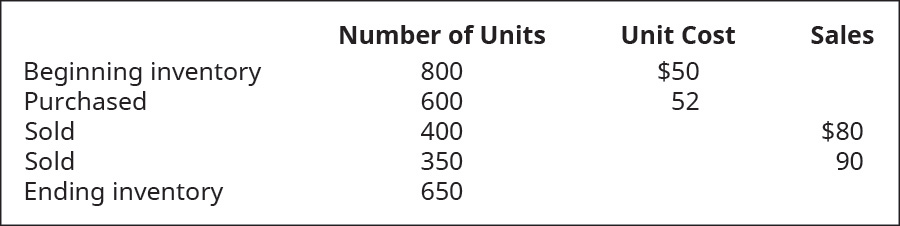# Calculate the cost of goods sold dollar value for A67 Company for the month, considering the following transactions under three different cost allocation methods and using perpetual inventory updating. Provide calculations for weighted average (AVG).FindFindarrow_forward

### Principles of Accounting Volume 1

19th Edition
OpenStax
Publisher: OpenStax College
ISBN: 9781947172685

#### Solutions

Chapter
SectionFindFindarrow_forward

### Principles of Accounting Volume 1

19th Edition
OpenStax
Publisher: OpenStax College
ISBN: 9781947172685
Chapter 10, Problem 10EA
Textbook Problem
14 views

## Calculate the cost of goods sold dollar value for A67 Company for the month, considering the following transactions under three different cost allocation methods and using perpetual inventory updating. Provide calculations for weighted average (AVG).To determine

Concept introduction:

Perpetual Inventory System:

It records and updates the inventory after each and every transaction. The inventory balance is updated and kept up to date.

Cost of goods sold = Beginning Inventory + Purchases − Ending Inventory

To calculate:

The cost of goods sold using the weighted average perpetual method.

### Explanation of Solution

The cost of goods sold using the weighted average perpetual methodis calculated as follows:

Weighted average cost per unit = ((800×50) +(600×52))(

### Still sussing out bartleby?

Check out a sample textbook solution.

See a sample solution

#### The Solution to Your Study Problems

Bartleby provides explanations to thousands of textbook problems written by our experts, many with advanced degrees!

Get Started